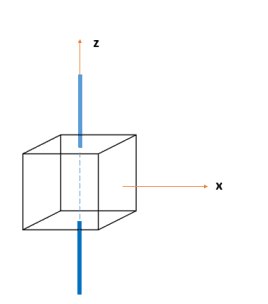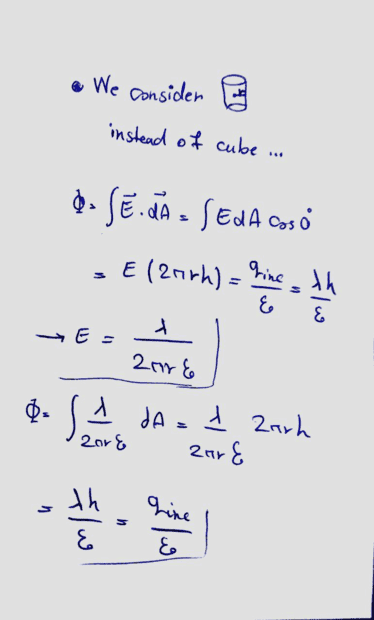# Electrical flux passing through the cube

• MatinSAR

#### MatinSAR

Homework Statement
Consider a rod with an electric charge density λ that passes through the center of the cube. Prove that the electric flux passing through this cube is equal to = q / ε0
Relevant Equations
Gauss's lawPicture for better understanding.I want to know how to solve this problem without using cylindrical. I mean how can we solve this using cube and its sides.
Thanks.

MatinSAR said:
Relevant Equations:: Gauss's law

I want to know how to solve this problem without using cylindrical. I mean how can we solve this using cube and its sides.

##\ ##

•MatinSAR
BvU said:

##\ ##
Thank you ... but what do you mean by relevant equation?

Does your relevant equation worry about the shape of the surface ?

On the other hand,
Prove that the electric flux passing through this cube is equal to = q / ε0
could be interpreted as an invitation to show that for this case the gauss identity holds. In that case you could actually integrate the flux: you know the electric field (right?) and can benefit from symmetry.

##\ ##

Last edited:
•MatinSAR
BvU said:
Does your relevant equation worry about the shape of the surface ?
I guess no it doesn't ... Thank you for your help ...

•berkeman